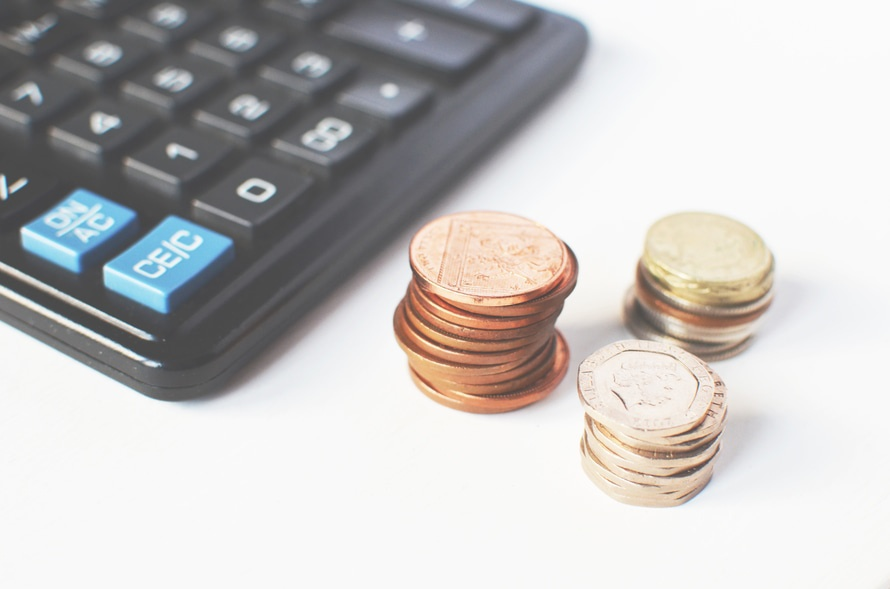# What is a Pre-money and Post-money Valuation?

By Venture CapitalWhen a startup raises capital, valuation is main economic term that must be tackled. The two main ways valuation is expressed in venture capital financings are what’s known as the “pre-money valuation” and the “post-money valuation”.

The startup’s valuation immediately before the venture capital investment is called “pre-money valuation” while the startup’s valuation immediately after the venture capital financing is closed is called the “post-money valuation.”

Equation (1) below explains how to calculate the pre-money valuation.  But sometimes a startup is not given the post-money valuation figure from an investor and therefore can’t make the easy pre-money valuation determination via Equation (1) below. Rather, the investor will tell the startup (a) how much they are investing and (b) what percentage they want — this is where Equation (2) below comes into play.  Then after solving Equation (2) first, then the startup founders can solve Equation 1 and determine the pre-money valuation being offered.

Let’s walk through the formulas first:

Pre-money Valuation and Post-money Valuation Equations

(1) Pre-money Valuation = Post-money valuation – Venture Capital Investment

(2) Post-money Valuation = Venture Capital Investment/Venture Capital Fund Ownership Percentage

Note that to you can determine share price by the following equation:

(3) Share Price = Pre-money Valuation/Number of Pre-money shares

(Pre-money shares is the number of shares outstanding immediately prior to the new venture capital investment.)

Next, you can also determine how many shares to issue the venture capital firm by this equation:

(4) New Shares Issued = Venture Capital Investment/Share Price

Now let’s walk through some examples:

Pre-money Valuation and Post-money Valuation Examples

You Might Also Like:  Guy Kawasaki: The Art of Raising Venture Capital, Part Three

Example 1

Let’s say Google’s new venture fund comes to you and offers to invest \$3MM into your startup for 30% of the company. Plugging the numbers into equation (2) above, we get:

Post-money valuation = \$3MM/.30 = \$10MM

Thus, to calculate pre-money valuation, we use equation (1) as we now know the post-money valuation and the investment amount:

Pre-money valuation = \$10MM – \$3MM = \$7MM

Example 2

Now let’s say a venture capital firm offers your startup company a \$4MM investment at a \$6MM pre-money. To determine how much your startup would give up in exchange for the \$4MM, we use equation (1) and get:

\$6MM = Post-money valuation – \$4MM, and solving for Post-money valuation (Post-money = Pre-money + Investment) gives us \$10MM

Next, we use equation (2) to find the Venture Capital firm’s percentage:

\$10MM = \$4MM/Venture Capital Firm Ownership Percentage (VCFOP), solving for VCFOP (VCFOP = \$4MM/\$10MM) we get 40%.

Conclusion

The two main concepts of valuation for startups are related, but they are often mistakenly interchanged.  If you bookmark this page, you can be sure to know what your pre-money and post-money valuation is when a prospective investor gives you the variables for the above equations.

### Startup Law doesn’t have to be a confusing maze. The practical knowledge in "Acceleration: What All Entrepreneurs Must Know About Startup Law" will help you make the smart decisions to protect your startup and its future. Available in ebook and hardcover.Tagged under: